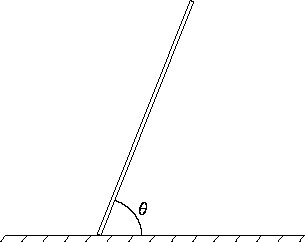Physics 321 -- Spring 2006

Homework #5, due at beginning of class Wednesday Feb. 22

Suggested reading: Marion and Thornton Chapter 3, sections 3.1 – 3.6 and Appendix C.

1.         [3pts] The force on a particle of mass M is equal to  - K p2   where p is the momentum and K is a constant.
(a) Find the velocity v as a function of time, given that v = v0 at time t = 0.
(b) Find the position x as a function of time, given that x = x0 at time t = 0.

2.         [6pts] A stick of length b has mass per unit length sigma = K (b2 - s2), where K is a constant and s is the distance measured along the stick starting at the bottom end. The stick is falling to the floor. The end on the floor is not slipping. Let  theta  be the angle with respect to the floor.(a) Find (1) the kinetic energy, (2) the potential energy, (3) the angular momentum about the point of contact with the floor, (4) the torque about the point of contact with the floor.
(b) Use your results for (3) and (4) to find the equation of motion.
(c) Use your results for (1) and (2) to find the equation of motion and show that your result agrees with part (b).

3.         [3pts] Marion & Thornton: problem 3-1 (Same in 4th edition). Give your answers in SI (SI=Standard International = kg,meter,sec) units, even though the numerical values in this problem are specified in old-fashioned cgs units.

4.         [3pts] Marion & Thornton, problem 3-2 (Same in 4th edition). The term "decrement" used in part (c) is defined in the text.

5.         [3pts] Marion & Thornton, problem 3-3 (Same in 4th edition). Again give your answers in SI units.

6.         [3pts] Marion & Thornton, problem 3-9 (Same in 4th edition).

(Last updated 2/15/2006.)